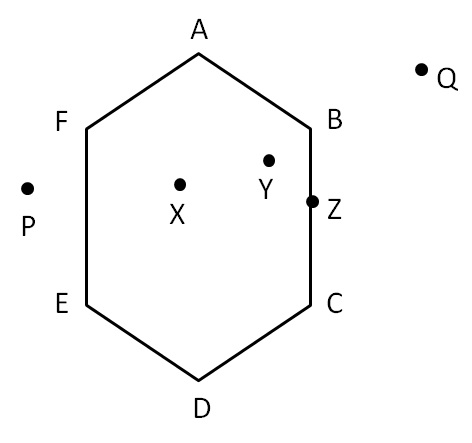Here,

• Point X, Y are in interior of polygon
• Point P, Q are in exterior of polygon
• Point Z is on the polygon

1. Chapter 3 Class 8 Understanding Quadrilaterals
2. Concept wise
3. Polygons

Polygons

Chapter 3 Class 8 Understanding Quadrilaterals
Concept wise